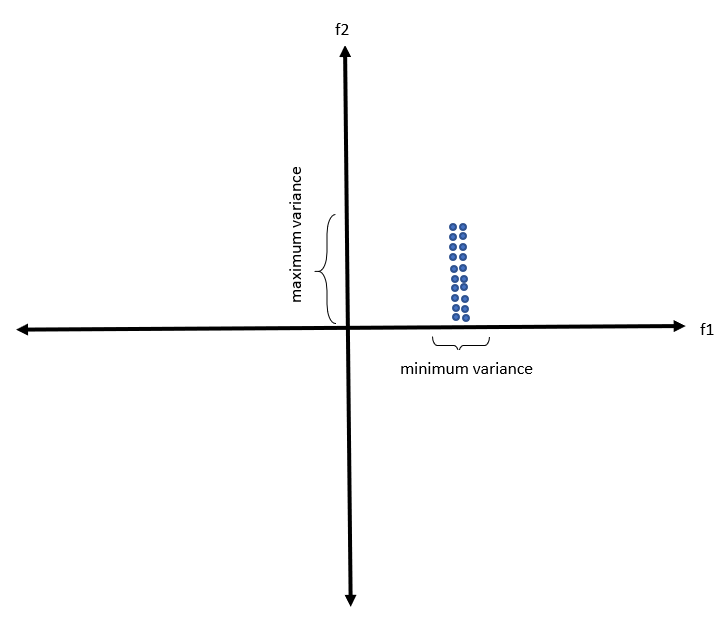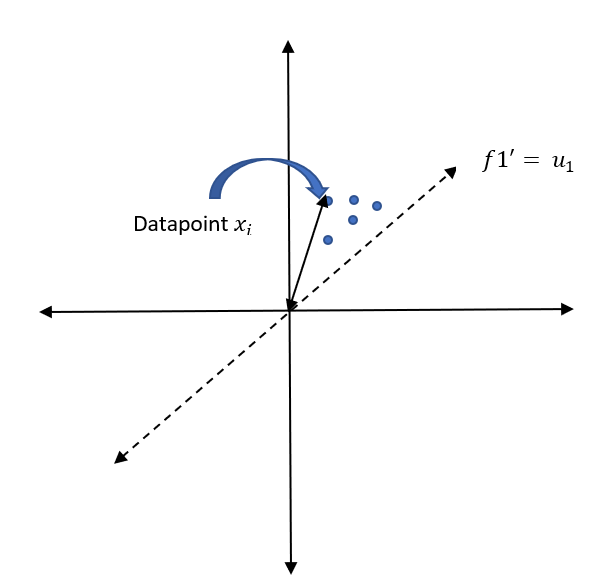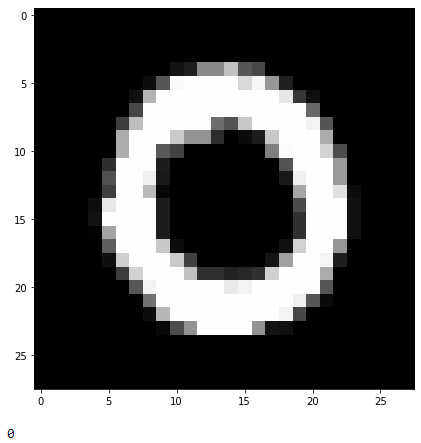# PCA for Visualization and Dimension Reduction….

1. PCA intuition (geometric)
2. Column standardization
3. Optimization problem
4. Calculation of covariance matrix
5. Eigen values and Eigen vectors
6. Interpretation of Eigen values
7. Summary
8. Implementation of PCA from scratch
9. Implementation of PCA using scikit learn
10. Limitations of PCA
11. Conclusion
12. References

## PCA intuition:

Principal component analysis (PCA) is the process of computing the principal components and using them to perform a change of basis on the data, sometimes using only the first few principal components and ignoring the rest. Let us consider a 2-D dataset with feature1(f1) in the x-axis and feature2(f2) in the y-axis.Data points on f1 and f2 axis

## Column Standardization:

Now, let us standardize the data points.

## Optimization problem:

We now want to find the direction u1 such that the variance of Xi’s projected onto f1' is maximized. Let us understand this statement by looking at the picture below.data point projected on the plane

## Calculation of co-variance matrix:

As the data column is standardized, hence the second term is zero. So, our final equation becomes,

## Eigen values and Eigen vectors:

Using the power of Lagrange multipliers, we can rewrite the above equation as

## Interpretation of eigenvalues(ƛ):

Let us understand the geometric interpretation of eigenvalues by looking at different cases.

## Summary:

1. Perform column standardization.
2. Calculate the covariance matrix.
3. Compute the eigenvalues and eigenvectors from the covariance matrix.
4. Sort these eigenvalue pairs in descending order of magnitude.
5. Select the top eigenvalues which retain maximum variance.
6. Perform data transformation original data by using the eigenvectors corresponding to top eigenvalues.

## Implementation of PCA from scratch:

Lets work with digit recognizer dataset. It has 42000 rows and 784 columns. Let me break this dataset. Say, you have dataset of digit images and classes ranging from 0–9. Each image has dimension of 28 x 28 (784). I hope you can correlate now. Each row contains a label from 0–9 and 784 pixel values as a column.

`#importing the librariesimport numpy as npimport pandas as pdimport matplotlib.pyplot as plt# reading the datad0 = pd.read_csv('train.csv')# save the labels into a variable l.labels = d0['label']# Drop the label feature and store the pixel data in d.data = d0.drop("label",axis=1)# Finding the size of dataprint(data.shape)print(labels.shape)`
`# display or plot a number.plt.figure(figsize=(7,7))idx = 1# reshape from 1d to 2d pixel arraygrid_data = data.iloc[idx].to_numpy().reshape(28,28)plt.imshow(grid_data, interpolation = "none", cmap = "gray")plt.show()print(labels[idx])`The value stored in the first index
`# Data-preprocessing: Standardizing the datafrom sklearn.preprocessing import StandardScalerstandardized_data = StandardScaler().fit_transform(data)print(standardized_data.shape)(42000, 784)`
`#find the co-variance matrix which is : A^T * Asample_data = standardized_data# matrix multiplication using numpycovar_matrix = np.matmul(sample_data.T , sample_data)print ( "The shape of variance matrix = ", covar_matrix.shape)The shape of variance matrix =  (784, 784)`
`from scipy.linalg import eigh# the parameter 'eigvals' is defined (low value to heigh value) # eigh function will return the eigen values in asending order# this code generates only the top 2 (782 and 783) eigenvalues.values, vectors = eigh(covar_matrix, eigvals=(782,783))print("Shape of eigen vectors = ",vectors.shape)# converting the eigen vectors into (2,d) shape for easyness of further computationsvectors = vectors.Tprint("Updated shape of eigen vectors = ",vectors.shape)Shape of eigen vectors =  (784, 2)Updated shape of eigen vectors =  (2, 784)`
`import matplotlib.pyplot as pltnew_coordinates = np.matmul(vectors, sample_data.T)print (" resultant new data points' shape ", vectors.shape, "X", sample_data.T.shape," = ", new_coordinates.shape)resultant new data points' shape  (2, 784) X (784, 42000)  =  (2, 42000)`
`import pandas as pd# appending label to the 2d projected datanew_coordinates = np.vstack((new_coordinates, labels)).T# creating a new data frame for ploting the labeled points.dataframe = pd.DataFrame(data=new_coordinates, columns=("1st_principal", "2nd_principal", "label"))print(dataframe.head())1st_principal  2nd_principal  label0      -5.226445      -5.140478    1.01       6.032996      19.292332    0.02      -1.705813      -7.644503    1.03       5.836139      -0.474207    4.04       6.024818      26.559574    0.0`
`import seaborn as snsn.FacetGrid(dataframe, hue="label", size=6).map(plt.scatter, '1st_principal', '2nd_principal').add_legend()plt.show()`

## Implementation of PCA using Scikit-learn:

Lets perform the same operation with Scikit-learn

`# initializing the pcafrom sklearn import decompositionpca = decomposition.PCA()# configuring the parameteres# the number of components = 2pca.n_components = 2pca_data = pca.fit_transform(sample_data)# pca_reduced will contain the 2-d projects of simple dataprint("shape of pca_reduced.shape = ", pca_data.shape)# attaching the label for each 2-d data point pca_data = np.vstack((pca_data.T, labels)).T# creating a new data fram which help us in ploting the result datapca_df = pd.DataFrame(data=pca_data, columns=("1st_principal", "2nd_principal", "label"))sn.FacetGrid(pca_df, hue="label", size=6).map(plt.scatter, '1st_principal', '2nd_principal').add_legend()plt.show()`
`# PCA for dimensionality redcution (non-visualization)pca.n_components = 784pca_data = pca.fit_transform(sample_data)percentage_var_explained = pca.explained_variance_ / np.sum(pca.explained_variance_);cum_var_explained = np.cumsum(percentage_var_explained)# Plot the PCA spectrumplt.figure(1, figsize=(6, 4))plt.clf()plt.plot(cum_var_explained, linewidth=2)plt.axis('tight')plt.grid()plt.xlabel('n_components')plt.ylabel('Cumulative_explained_variance')plt.show()`

Case1:

## Conclusion:

PCA is simple algorithm which assumes linearly correlated dataset and convert high dimension data into low dimension. It is observed that PCA performs good during conversion of data but not in visualization. However, there are better algorithm like T-SNE, which assumes non- linear dataset and preserves local structure of the dataset.

--

--